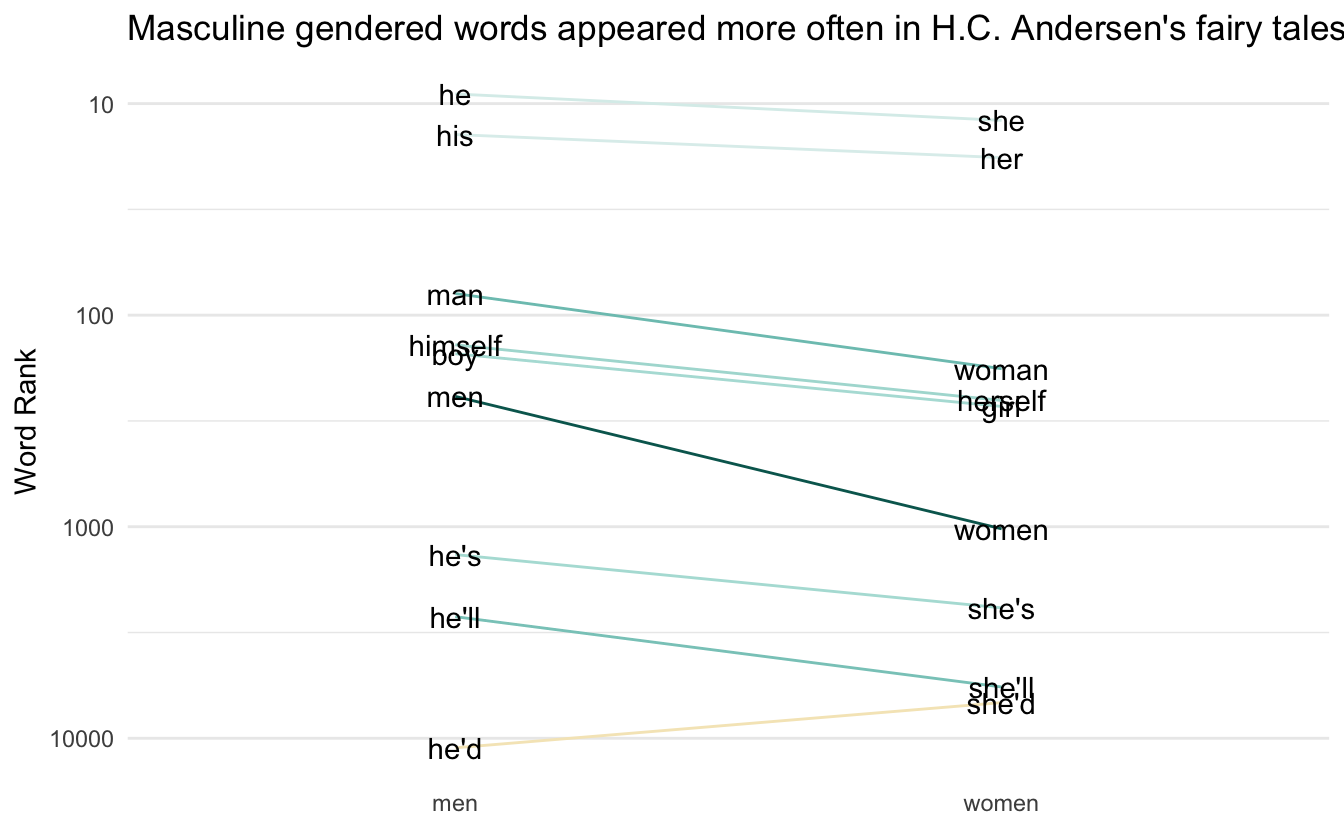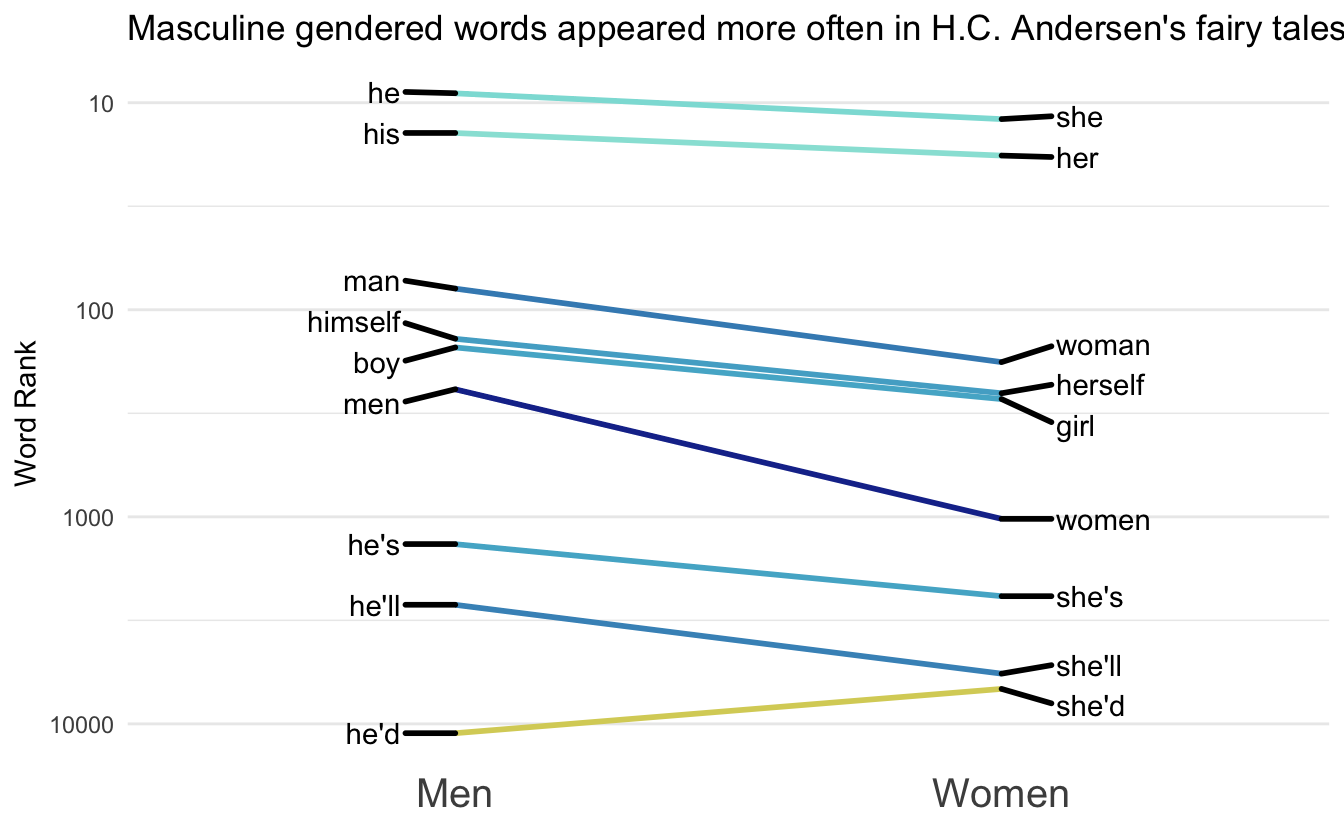# Word Rank Slope Charts

I have been working on visualizing how different kinds of words are used in texts and I finally found a good visualization style with the slope chart. More specifically I’m thinking of two groups of paired words.

## Packages 📦

``````library(tidyverse)
library(hcandersenr)
library(tidytext)
library(paletteer)
library(ggrepel)``````

## Minimal Example 1️⃣

First I’ll walk you through a minimal example of how the chart is created. Afterward, I have created a function to automate the whole procedure so we can quickly iterate. We start by an example of gendered words in fairly tales by H.C. Andersen using the hcandersenr package. We start by generating a data.frame of paired words. This is easily done using the `tribble()` function.

``````gender_words <- tribble(
~men, ~women,
"he", "she",
"his", "her",
"man", "woman",
"men", "women",
"boy", "girl",
"he's", "she's",
"he'd", "she'd",
"he'll", "she'll",
"himself", "herself"
)``````

Next, we are going to tokenize and count the tokens in the corpus,

``````ordered_words <- hcandersen_en %>%
unnest_tokens(word, text) %>%
count(word, sort = TRUE) %>%
pull(word)``````

Next, we are going to get the index for each word, which we will put on a log scale since it will be easier to visualize. Next, we will calculate a slope between the points and add the correct labels.

``````gender_words_plot <- gender_words %>%
mutate(male_index = match(men, ordered_words),
female_index = match(women, ordered_words)) %>%
mutate(slope = log10(male_index) - log10(female_index)) %>%
pivot_longer(male_index:female_index) %>%
mutate(value = log10(value),
label = ifelse(name == "male_index", men, women)) %>%
mutate(name = factor(name, c("male_index", "female_index"), c("men", "women")))``````

Next, we are going to manually calculate the limits to make sure a diverging color scale will have the colors done directly.

``limit <- max(abs(gender_words_plot\$slope)) * c(-1, 1)``

Lastly, we just put everything into ggplot2 and voila!!

``````gender_words_plot %>%
ggplot(aes(name, value, group = women, label = label)) +
geom_line(aes(color = slope)) +
scale_y_reverse(labels = function(x) 10 ^ x) +
geom_text() +
guides(color = "none") +
scale_color_distiller(type = "div", limit = limit) +
theme_minimal() +
theme(panel.border = element_blank(), panel.grid.major.x = element_blank()) +
labs(x = NULL, y = "Word Rank") +
labs(title = "Masculine gendered words appeared more often in H.C. Andersen's fairy tales")``````## Make it into a function ✨

This function is mostly the same as the code you saw earlier. Main difference is using `.data` from rlang to generalize. The function also includes other beautifications such as improved themes and theme support with paletteer.

``````plot_fun <- function(words, ref, palette = "scico::roma", ...) {

names <- colnames(ref)

ordered_words <- names(sort(table(words), decreasing = TRUE))

plot_data <- ref %>%
mutate(index1 = match(.data[[names]], ordered_words),
index2 = match(.data[[names]], ordered_words)) %>%
mutate(slope = log10(index1) - log10(index2)) %>%
pivot_longer(index1:index2) %>%
mutate(value = log10(value),
label = ifelse(name == "index1",
.data[[names]],
.data[[names]]),
name = factor(name, c("index1", "index2"), names))

limit <- max(abs(plot_data\$slope)) * c(-1, 1)

plot_data %>%
ggplot(aes(name, value, group = .data[[names]], label = label)) +
geom_line(aes(color = slope), size = 1) +
scale_y_reverse(labels = function(x) round(10 ^ x)) +
geom_text_repel(data = subset(plot_data, name == names),
aes(segment.color = slope),
nudge_x       = -0.1,
segment.size  = 1,
direction     = "y",
hjust         = 1) +
geom_text_repel(data = subset(plot_data, name == names),
aes(segment.color = slope),
nudge_x       = 0.1,
segment.size  = 1,
direction     = "y",
hjust         = 0) +
scale_color_paletteer_c(palette,
limit = limit,
aesthetics = c("color", "segment.color"),
...) +
guides(color = "none", segment.color = "none") +
theme_minimal() +
theme(panel.border = element_blank(),
panel.grid.major.x = element_blank(), axis.text.x = element_text(size = 15)) +
labs(x = NULL, y = "Word Rank")
}``````

Now we can recreate the previous chart with ease

``````ref <- tribble(
~Men, ~Women,
"he", "she",
"his", "her",
"man", "woman",
"men", "women",
"boy", "girl",
"he's", "she's",
"he'd", "she'd",
"he'll", "she'll",
"himself", "herself"
)

words <- hcandersen_en %>%
unnest_tokens(word, text) %>%
pull(word)

plot_fun(words, ref, direction = -1) +
labs(title = "Masculine gendered words appeared more often in H.C. Andersen's fairy tales")
## Warning: Ignoring unknown aesthetics: segment.colour

## Warning: Ignoring unknown aesthetics: segment.colour``````